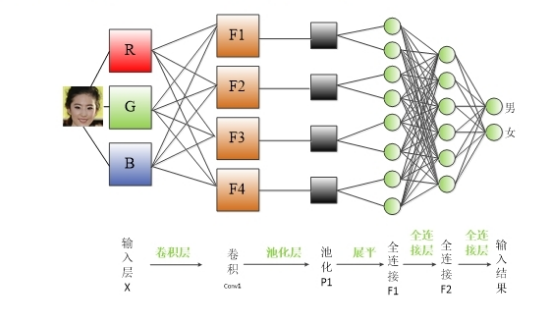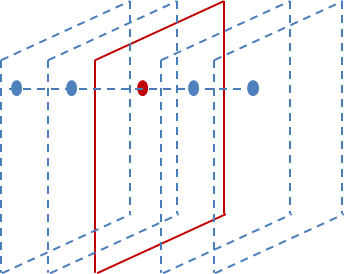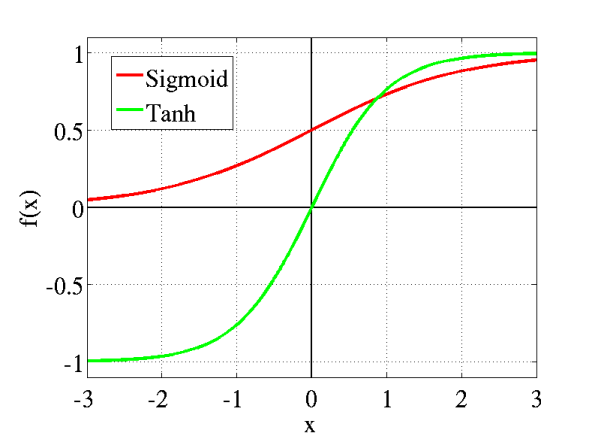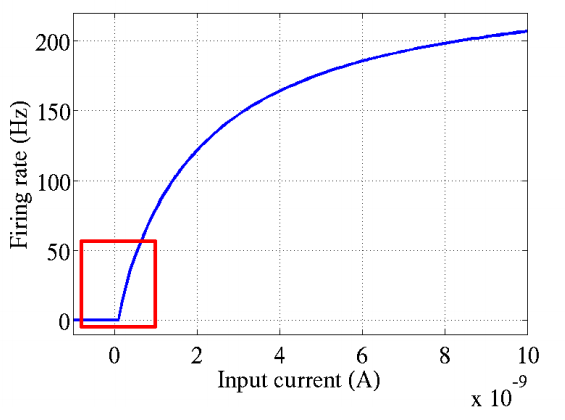# 深度学习：卷积神经网络与图像识别基本概念

## 三 网络的中段

### 3.1 卷积神经网络的基本组成### 3.4 CCCP

CCCP层的输入是$x\epsilon R^{W\times H\times C}$，输出是$y\epsilon W\times H\times C$。特征层的分辨率保持不变，但是通道数有所改变。其定义为： $y^{c_0}_{u,v}=\sum_cx^c_{u,v}w^{c,c_0}$ 等效于卷积核为 1x1的卷积层。 CCCP 层相当于在多个全连接层，每一个全连接将信号从 C 维度映射为$C_0$维度。

### 3.6 Dropout层

Dropout层的输入为$x\epsilon R^{W\times H\times C}$。这里并不要求输入是三维的信号，任意可能的维度都是可以。Dropout 同样是针对每一个数据进行操作。输出 y 与输入的大小一致。在网路进行训练的时候，对于输入的每一个数值 $x_i$，按照概率 p 设置为 0，否则保留。数学形式可以写为: $y_i=\epsilon x_i$ 其中 $\epsilon$ 是随机变量，并且满足 $\epsilon = 0$的概率为 p，$\epsilon = 1$ 的概率为 1 − p。实际中，概率 p 往往设置 为 1。

Dropout层的引入主要是为了减少过拟合的问题，减少不同参数的耦合性。

### 3.8 局部响应归一化(LRN)

LRN层做的事是对当前层的输出结果做平滑处理。下图是示例:前后几层（对应位置的点）对中间这一层做一下平滑约束。

### 3.9 批归一化层(Batch Normalization)

• 可以选择比较大的初始学习率,此算法有快速训练收敛的特性。
• 可以不用考虑过拟合中的 Dropout ,L2正则项选择问题，采用BN之后可以移除这两个参数，或者可以选择更小的L2正则约束参数了，因为BN具有提高网络泛化能力的特性。
• 不再需要局部响应归一化层，因为BN本身就是一个归一化网络层。
• 可以完全打乱训练数据，防止每批训练时某些样本经常被挑选到。

## 四 网络的末端

### 4.1 Softmax回归

$Z_i =\frac{exp\{v_i\}}{\sum_j exp\{v_j\}}$ 然后通过交叉熵定义损失值，也就是： $y = -log(Z_k)$ 该损失函数主要应用与单类别分类问题中。 下图是$softmax$函数的坐标轴图像:从数学上来看，非线性的Sigmoid函数对中央区的信号增益较大，对两侧区的信号增益小，在信号的特征空间映射上，有很好的效果。 从神经科学上来看，中央区酷似神经元的兴奋态，两侧区酷似神经元的抑制态，因而在神经网络学习方面，可以将重点特征推向中央区，将非重点特征推向两侧区。

### 4.2 近似神经激活函数:Softplus&ReLu

2001年，神经科学家Dayan、Abott从生物学角度，模拟出了脑神经元接受信号更精确的激活模型，该模型如下图所示: